# HC Verma Solutions Class 12 Chapter 7 Electric Field And Potential

HC Verma Solutions Class 12 Chapter 7 Electric Field and Potential is a very useful study material if students are preparing for exams especially competitive ones like JEE, NEET amongst others. The solutions help students to not only increase their understanding of concepts but it will also help them learn the right way of solving problems. The solution provided here further contain all the answers to the exercises given in chapter 7 of the HC Verma book vol 2.

Students can make use of the HC Verma solutions and study productively for the exams. They can also use the solutions to complete assignments or simply practice questions to enhance their preparation level. The solutions that we have provided here have been drafted by physics experts and students can refer them without having any doubt.

## Electric Field And Potential Key Topics

Some of the important topics covered are;

• Definition of an Electric Charge
• Coulomb’s Law
• Electric Field
• Lines of Electric Force
• Electric Potential Energy
• Electric Potential
• Electric Dipole
• Conductors, Insulators, Semiconductors

## Class 12 Important Questions In Chapter 7

1. If we take a point charge from point A to point B in an electric field does the work done depend on the path of the charge? What happens when a charge is placed at rest in an electric field?

2. Both insulators and conductors have electrons in the same order. Write down the basic difference between a conductor and an insulator?

3. If we increase the separation between the two charges what will happen to the electric potential energy of the charges?

(a) remains the same (b) decreases (c) increases (d) may increase or decrease.

4. When an electric dipole is placed in a uniform electric field what happens to the net electric force on the dipole?

(a) it is zero (b) can never be zero (c) depends on the orientation of the dipole (d) depends on the strength of the dipole.

5. Can you pick out the quantities from the following that do not depend on the choice of zero potential or zero potential energy?

(a) the potential energy of a two-charge system
(b) change in potential energy of a two-charge system
(c) the potential difference between two points
(d) the potential at a point

Important Formulas:

1. Formula for Electrostatic force,

By Coulomb’s law: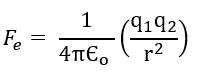………(A)

Where,

Єo is the permittivity of vacuum

q1, q2 is the magnitude of the charges on the charge particles

r is the distance between the two charge particles.

2. By Newton’s law of gravitation, the gravitational force: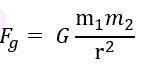……….(B)

Where G is the Gravitational constant

m1 and m2 are the magnitude of charges

r is the distance of separation between the masses.

## HC Verma Solutions Vol 2 Electric Field and Potential Chapter 7

Question 1: Find the dimensional formula of ϵ0.

Solution:

Dimensions of F = [MLT−2]

Dimensions of q = [AT]

Dimensions of r = [L]

Now, using Equation (A), we have

Dimensional of ϵo = [AT][AT]/[MLT−2][L2] = [M-1L-3T4A2]

Question 2: A charge of 1.0 C is placed at the top of your college building and another equal charge at the top of your house. Take the separation between the two charges to be 2.0 km. Find the force exerted by the charges on each other. How many times of your weight is this force?

Solution:

q1 = q2 = 1.0 C (Given)

Let “r” be the distance between the charges.

=> r = 2 km = 2 x 103 m

By Coulomb’s law,

using equation (A)

F = 9 x 109 x (1×1)/(2×103)2 = 2.25 x 103 N

Again, we know W = mg

Let the mass of my body, m = 70 kg.

W = 70 x 9.8 = 686 N

Now, on dividing the Electrostatic force and the body weight, we have

F/W = [2.25 x 103]/686 = 3.3 (approx)

The electric force between the two charges is 3.3 times my weight.

Question 3: At what separation should two equal charges, 1.0 C each, be placed so that the force between them equals the weight of a 50 kg person?

Solution:

q1 = q2 = 1.0 C (Given)

Let “m” be the mass of the person and “w” be weight.

=> m = 50 kg and w = mg = 50 x 9.8 = 490 N

By Coulomb’s law,

using equation (A)

Here Fe = w = 490 N

On rearranging and substituting the given values, we get

r = 4.3 x 103 m (approx)

Question 4: Two equal charges are placed at a separation of 1.0 m. What should be the magnitude of the charges so that the force between them equals the weight of a 50 kg person?

Solution:

q1 = q2 = q (Given)

Let “m” be the mass of the person and “w” be weight.

=> m = 50 kg and w = mg = 50 x 9.8 = 490 N

Two equal charges are placed at a separation of 1.0 m, so r = 1.0 m

By Coulomb’s law,

using equation (A)

Here Fe = w = 490 N

On rearranging and substituting the given values, we get

q = 2.3 x 10-4 C (approx)

Question 5: Find the electric force between two protons separated by a distance of 1 fermi (1 fermi = 10–15 m). The protons are a nucleus remain at a separation of this order.

Solution:

q1 = q2 = 1.6×10-19 C (Given)

Two equal charges are placed at a separation, r = 1 fermi = 10-15 m

By Coulomb’s law,

using equation (A)

Here Fe = k [q1q2]/r2

= [9×109x1.6×10-19x1.6×10-19]/(10-15)2

Fe = 230 N (approx)

Question 6: Two charges 2.0 × 10–6 C and 1.0 × 10–6 C are placed at a separation of 10 cm. Where should a third charge be placed such that it experiences no net force due to these charges?

Solution:

q1 = 1.0 × 10-6 C and q2 = 2.0×10-6 C (Given)

Distance between the charge, r = 10 cm

Let “q” be the third charge and placed at a distance of x cm from the charge q1.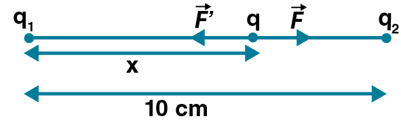By Coulomb’s law,

using equation (A)

Force on charge q due to charge q1

F1 = [9×109x2x10-6xq]/x2

Again, Force on charge q due to charge q2

F2 = [9×109x10-6xq]/(10-x)2

According to question: F1 = F2

On equating above equations and solving, we get

=> x2 = 2(10 – x)2

=> x2 – 40x + 200 = 0

=>x = 20 + 10√2 or x = 20 – 10√2

As x > 0. The correct option is x = 20 + 10√2

The charge q should be placed 5.9 cm from q1.

Question 7: Suppose the second charge in the previous problem is –1.0 × 10–6 C. Locate the position where a third charge will not experience a net force.

Solution:

q1 = -1.0×10-6 C, q2 = 2.0×10-6 C (given)

3rd charge can not be placed between q1 and q2 as both the charges are opposite in nature.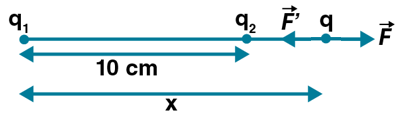By Coulomb’s law,

using equation (A)

Force on charge q due to q1:

F1 = [9×109x2x10-6xq]/x2

Force on charge q due to q2

F2 = [9×109x10-6xq]/(x-10)2

According to question: F1 = F2

On equating above equations and solving, we get

=> x2 = 2(x-10)2

Or x = 5.86 and 34.1

Since x > 0, This implies, x = 34.14 cm

Thus, q should be placed 34.1 cm from the larger charge on the side of the smaller charge.

Question 8: Two charged particles are placed at a distance 1.0 cm apart. What is the minimum possible magnitude of the electric force acting on each charge?

Solution:

For the electric force to be minimum, the magnitude of charge on the particles must be minimum.

Magnitude of charges, q1 = q2 = 1.6×1019 C

Distance between the charged particles, r = 1 cm = 0.01 m

By Coulomb’s law,

using equation (A)

Fe = [9×109x1.6×10-19x1.6×1019]/(0.01)2

= 2.3 x 10-24 N (approx)

Question 9: Estimate the number of electrons in 100 g of water. How much is the total negative charge on these electrons?

Solution:

mass of water = m = 100 g

Moles of water in 100g of water = (100/18) mol

[Molar mass of water = 18 g/mol]

Number of electrons in 1 molecules of water = (2×1)+8 = 10

Number of electrons in 6.023×1023(Avogadro’s number) molecules of water = 6.023×1023 x 10 = 6.023×1024

=> Number of electrons in 18 g of water = 6.023×1024

so, Number of electrons in 100 g of water = [6.023×1024]/18 x 100 = 3.34 x 1025

We know, Negative charge on one electron = 1.6 x 10-19 C

Therefore, total charge = 3.34 x 1025 x 1.6 x 10-19 = 5.35 x 106 C (approx)

Question 10: Suppose all the electrons of 100g water are lumped together to form a negatively charged particle and all the nuclei are lumped together to form a positively charged particle. If these two particles are placed 10.0 cm away from each other, find the force of attraction between them. Compare it with your weight.

Solution:

Mass of water = m = 100 g

Moles of water in 100g of water = (100/18) mol

[Molar mass of water = 18 g/mol]

Number of electrons in 1 molecules of electrons = 10 electrons x 6.023×1023 mol-1

Number of electrons in 100 g of water = 10 x 6.023 x 1023 x 100/18 = 3.35 x 1025 electrons

We know, Negative charge on one electron = 1.6 x 10-19 C

Therefore, total negative charge = 3.35 x 1025 x 1.6 x 10-19 = 5.35 x 106 C (approx)

Now, we have q1 = -5.35 x 106 C and q2 = 5.35 x 106 C

and r = 10 cm = 0.1 m

By Coulomb’s law,

using equation (A)

The magnitude of electric force:

Fe = [9×109 x (5.35 x 106)2]/(0.1)2

= 2.57 x 1025 N (approx)

Let my body mass be 70 kg, then

Fw = mg = 70 x 9.8 = 686 N

Now, ratio is:

Fe/Fw = 3.7 x 1022

Question 11: Consider a gold nucleus to be a sphere of radius 6.9 fermi in which protons and neutrons are distributed. Find the force of repulsion between two protons situated at largest separation. Why do these protons not fly apart under this repulsion?

Solution:

Charge on each proton, q1 = q2 = 1.6 x 10-19 C

Let “d” be the diameter of the nucleus.

Radius of the sphere = R = 6.9 fermi

Largest Distance of Separation = Diameter of the nucleus = d = 2R = 13.8 fermi = 13.8 x 10-15 m

By Coulomb’s law, using equation (A), we get

F = 9 x 109 x [1.6×10-19)2/(2R) 2 = 1.2 N

The protons do not fly apart due to the strong nuclear force which is attractive and balances the repulsive electric force.

Question 12: Two insulating small spheres are rubbed against each other and placed 1 cm apart. If they attract each other with a force of 0.1N, how many electrons were transferred from one sphere to the other during rubbing?

Solution:

force = Fe = 0.1N

r = 1 cm = 0.01 m and e = 1.6 x 10-19

e = magnitude of charge on electron, r = Distance between the two spheres

According to question: q1 = q2 = ne

By Coulomb’s law, the electric force:

Fe = k(ne)2/r2

rearranging and substituting the values, we get,

n = 2 x 1011

Thus, about 2 x 1011 electrons are transferred.

Question 13: NaCl molecule is bound due to the electric force between the sodium and the chlorine ions when one electron of sodium is transferred to chlorine. Taking the separation between the ions to be 2.75 × 10-8 cm, find the force of attraction between them. State the assumptions (if any) that you have made.

Solution:

Distance between the ions = r = 2.75 x 10-10 m

Charge on Na+ ion = q = 1.6 x 10-19

Charge on Cl ion = -q = -1.6 x 10-19

By Coulomb’s law, the electric force:

Fe = kq2/r2

= [9×109x(1.6 x 10-19)2]/[2.75 x 10-10]2

= 3.05 x 10-9 N (approx)

Question 14: Find the ratio of the electric and gravitational forces between two protons.

Solution:

The electric force: Fe = kq2/r2 …(1)

The gravitational force, Fg = G (m2)/r2 …(2)

Now,

Fe/Fg = kq2/Gm2

Here k = 9x 109 NC-2m2; q = 1.6 x 10-19 C ; G = 6.67 x 10-11 N Kg-2m2 and m = 1.67 x 10-27 Kg

On putting all the values, we get

Fe/Fg = 1.23 x 1036 (approx)

Question 15: Suppose an attractive nuclear force acts between two protons which may be written as F = CE–kx/r2.

(a) Write down the dimensional formulae and appropriate SI units of C and κ.

(b) Suppose that κ = 1 fermi–1 and that the repulsive electric force between the protons is just balanced by the attractive nuclear force when the separation is 5 fermi. Find the value of C.

Solution:

force between two protons, F = CE–kx/r2 (Given)

(a) Dimensionless for k:

[k] = 1/[r] = [L-1]

SI unit of k is m.

Nuclear force of attraction: F = C ekr/r2

Here ekr is dimensionless quantity

[C] = [F][r2] = [MLT-2][L2]

=> [C] = [ML3T-2]

And, SI unit of C is Nm2

(b) Separation between the protons, r = 5fermi = 5 × 10-15 m (Given)

Charge on a proton, q = 1.6 × 10-19C.

The electric force between the two protons:

Fe = kq2/r2

Also, nuclear force between the protons:

Fn = C ekr/r2

These two forces balance each other, so Fe = Fn

=> kq2 = Ce-kr

Or kq2 /e-kr = C

=> C = [9×109x(1.6 × 10-19)2]/ e-1×5 = 3.4 x 10-26 Nm2 (approx.)

Question 16: Three equal charges, 2.0 × 10–6 C each, are held fixed at the three corners of an equilateral triangle of side 5 cm. Find the Coulomb force experienced by one of the charges due to the rest two.

Solution:

Let ABC be an equilateral triangle of each side “a”.

So, a = 5 cm = 0.05 m

Let the force due to charge at corner B, C be FB, FC.

And “q” be charge at each corner, q = 2.0 x 10-6.

By Coulomb’s law,

Fe = k(q1q2)/r2 = 1/4πЄo  [q1q2/r2]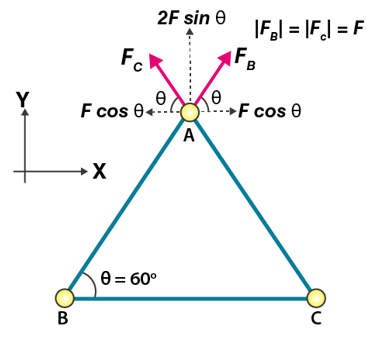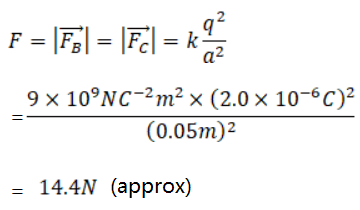From diagram, x and y components of FC and FB:

FC,x = F Cos 600 and FC,y = F Sin 600

Fb,x = F Cos 600 and Fb,y = F Sin 600

x’s are in the opposite direction and same in magnitude, so they cancel each other.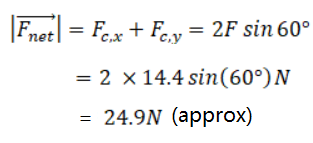Question 17: Four equal charges 2.0 × 10–6 C each are fixed at the four corners of a square of side 5 cm. Find the Coulomb force experienced by one of the charges due to the rest three.

Solution:

Let ABCD be a square of each side “a”.

So, a = 5 cm = 0.05 m

Let the force due to charge at corner B, C and D be FB, FC, FD.

And “q” be charge at each corner, q = 2.0 x 10-6.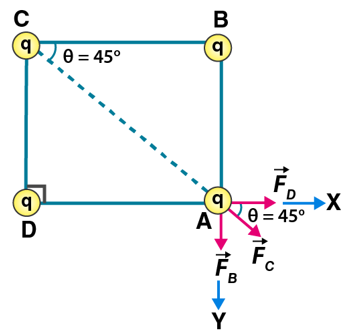From figure,

The length of diagonal = a√2 = 0.05√2 m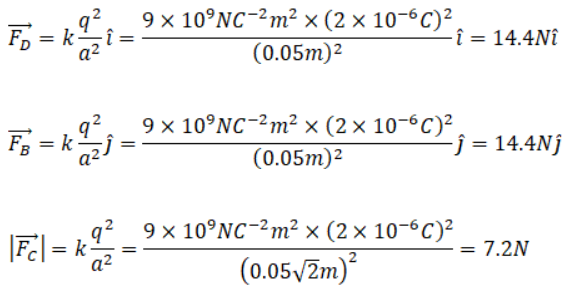Now,

Fx = |FC| cos θ = 7.2N cos(45o) = (7.2/√2) N

Fy = |FC| sin θ = 7.2N sin(45o) = (7.2/√2) N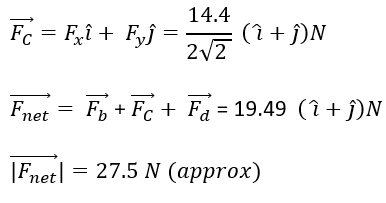Question 18: A hydrogen atom contains one proton and one electron. It may be assumed that the electron revolves in a circle of radius 0.53 angstrom(1 angstrom = 10-19 m and is abbreviated as Ao) with the proton at the center. The hydrogen atom is said to be in the ground sate in this case. Find the magnitude of the electric force between the proton and the electron of a hydrogen atom in its ground state.

Solution:

The magnitude of electric force:

[Using Coulomb’s law]

Fe = 1/4πЄo  [e2/r2]

Fe = 9 x 109 x [1.6×10-19]/[(0.53×10-10)2]

=> Fe = 8.2 x 10-8 N

Question 19: Find the speed of the electron in the ground state of a hydrogen atom. The description of ground state is given in the previous problem.

Solution:

The magnitude of electric force:

[Using Coulomb’s law]

Fe = 1/4πЄo  [e2/r2]

Fe = 9 x 109 x [1.6×10-19]/[(0.53×10-10)2]

=> Fe = 8.2 x 10-8 N

(using previous problem given)

Now,

Let FC be the centripetal force on the electron.

FC = mv2/r

As, electric force acts as centripetal force.

So , Fe = Fc

=>v = √(Fer)/m

=>v = √[8.2×10-8x0.53×10-10]/[9.11×10-31]

=>v = 2.18 x 106 ms-1

Question 20: Ten positively charged particles are kept fixed on the x-axis at points x = 10 cm, 20 cm 30 cm, ……, 100 cm. The first particle has a charge 1.0 × 10–8 C, the second 8 × 10–8 C, the third 27 × 10–8 C and so on. The tenth particle has a charge 1000 × 10–8 C. Find the magnitude of the electric force acting on a 1C charge placed at the origin.

Solution:

Charge on the ith particle = qi = i3 x 10–8C

Distance of ith particle from origin = r1 = 10i cm = 0.1i m

Magnitude of force on the 1C charge due to ith particle:

Fi = 1/4πЄo  [q1q2/r2] = 1/4πЄo  [1x 10-8xqi]/ri2]

=>Fi = 9000i N

Now, magnitude of net force: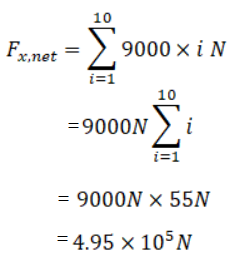Question 21: Two charged particles having charge 2.0 × 10-8 C each are joined by an insulating string of length 1m and the system is kept on a smooth horizontal table. Find the tension in the string.

Solution:

Let “q” be the charge on each particle that is, 2.0 × 10-8 C

And, Distance between the two charges = r = 1m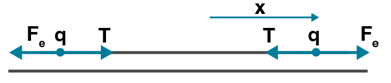The tension will adjust such that the net force is zero.

Using Coulomb’s law,

T = Fe = 1/4πЄo  [q2/r2]

=>T = [9×109x(2×10-8)]/ = 3.6 x 10-6 N

Question 22: Two identical balls, having a charge of 2.00 × 10-7 C and a mass of 100g, are suspended from a common point by two insulating strings each 50 cm long. The balls are held at a separation 5.0 cm apart and then released. Find

(a) the electric force on one of the charged balls

(b) the components of the resultant force on it along and perpendicular to the string

(c) the tension in the string

(d) the acceleration of one of the balls. Answers are to be obtained only for the instant just after the release.

Solution: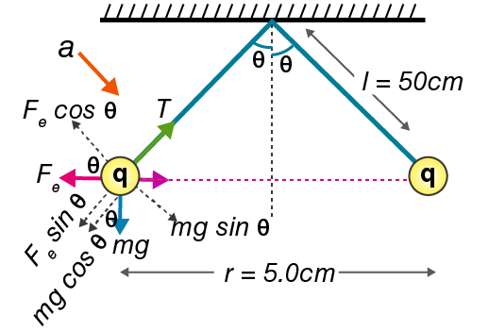(a)The magnitude of the electric force is:

Using Coulomb’s law,

Fe = 1/4πЄo  [q2/r2] = [(9×109)(2×10-7)2]/[0.05] = 0.144 N

Where, Distance between spheres = r = 5 cm = 0.05 m (given)

And, Magnitude of charge on each ball = q = 2×10-7 (given)

(b) From figure,

From figure, along the string, there is component of acceleration. So, y-component of force along y-axis is zero.

Now, consider the direction perpendicular to the string

Σ Fx = Fe cosθ – mg sinθ

= 0.144 x 0.99875 – 0.1 x 9.8 x 0.05

= 0.095 N (approx)

Therefore, component of net force perpendicular to the string is 0.095N and away from the other charge.

(c) We have, Σ Fy = T – Fe sinθ – mg cosθ = 0 from figure]

Or T = Fe sinθ + mg cosθ = 0.144 x 0.05 + 0.1 x 9.8 x 0.99875

= 0.986N (approx.)

(d) Apply Newton’s second law along the y-direction,

ΣFy = m ay

=> ay = 0.095/0.1 = 0.95 m/s2

And we have, ax = 0

So, net acceleration = a = 0.95 m/s2

Question 23: Two identical pith balls are charged by rubbing against each other. They are suspended from a horizontal rod through two strings of length 20 cm each, the separation between the suspension points being 5 cm. In equilibrium, the separation between the balls is 3 cm. Find the mass of each ball and the tension in the strings. The charge on each ball has a magnitude 2.0 × 10-8 C.

Solution: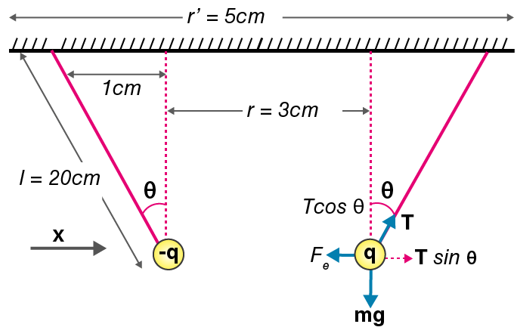Since r’ > r, the balls must be attracted to each other as both are oppositely charged.

At equilibrium, all forces must cancel out in accordance with newton’s first law. Hence net force = 0

The magnitude of the electric force:

Fe = 1/4πЄo  [q1q2/r2] [By Coulomb’s law]

Fe = [9×109x(2×10-8)2]/[0.032] = 0.004N

Since T sinθ = Fe and T cosθ = mg

=> T = 0.004/0.05 = 0.08 N and m = [0.08×0.99875]/9.8 = 0.008153 kg = 8.2 g

Therefore, Tension in each of the ropes is 0.08N and Mass of each of the balls is 8.2 grams.

Question 24: Two small spheres, each having a mass of 20g, are suspended from a common point by two insulating strings of length 40 cm each. The spheres are identically charged and the separation between the balls at equilibrium is found to be 4 cm. find the charge on each sphere.

Solution: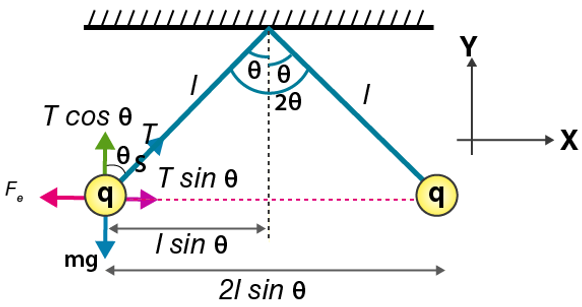Length of strings = l = 40cm = 0.4 m

Let “T” = magnitude of Tension, Fe = electric force between the spheres and q = magnitude of charge on each pitch ball.

Form figure, sinθ = 0.05 and cosθ = 0.99875

At equilibrium, all forces must cancel out in accordance with newton’s first law. [x-coordinate and y-coordinate of the force is zero.]

=> Fe = T sinθ and mg = T cosθ

Now,

T = mg/cosθ = [0.02 x 9.8]/[0.99875] = 0.1962 N (approx)

Also, T sinθ = 1/4πЄo  [q2/r2]

=> q2 = [0.1962×0.05x(0.04)2]/[9×109]

=>q = 4.17 x 10-8 C

Question 25: Two identical pith balls, each carrying a charge q, are suspended from a common point by two strings of equal length ℓ. Find the mass of each ball if the angle between the strings is 20 in equilibrium.

Solution:

At equilibrium,

Fe = T sinθ and mg = T cosθ

Let “r” be the distance between the two charges.

Sinθ = (r/2)/l or r = 2l sinθ

Using Coulomb’s law,

Fe = 1/4πЄo  [q2/(4l2sin2θ]

Or T sinθ = Fe = 1/4πЄo  [q2/(4l2sin2θ]

Or T = 1/4πЄo  [q2/(4l2sin3θ]

And m = (T cosθ)/g = 1/4πЄo  [q2/(4l2sin3θ] x cosθ/g

Or T = [q2cotθ]/[16πЄog l2sinθ]